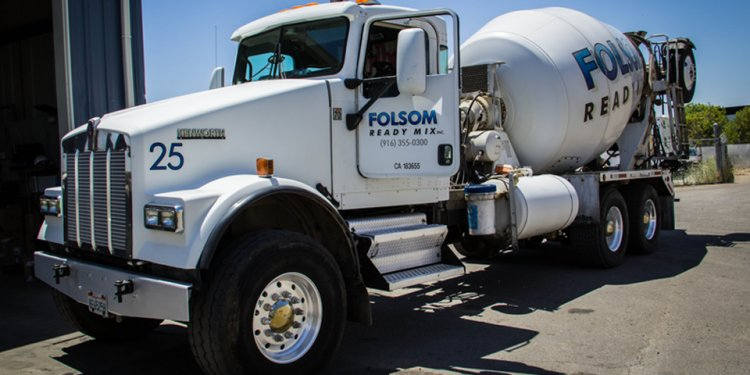# Concrete truck capacity yards

An order is received for 150 cubic yards of concrete. The contractor says he'll unload each truck, which carry 10 cubic yards of concrete, in 10 minutes using a pump. How many trucks should you assign to this project?

If we know the total round-trip time required for delivery, we can calculate the number of trucks that will be needed. The round-trip time is the amount of time, in minutes, required to load the truck, adjust the slump and clean the truck after loading, drive to the jobsite, unload, wash up, and, finally, return to the plant.

If we know this data the number of trucks can be calculated with the following equation:

= Number of Trucks Needed

This equation is accurate as long as the number of trucks calculated is less than the number of loads. Both numbers must be calculated and compared. The equation calculates the number of trucks to be batched for the project before the first truck has returned for its second load. After that point, no more trucks will be needed. The equation also assumes that, as one truck finishes unloading, another is just pulling up on the jobsite.

In the example, our order is for 150 cubic yards of concrete with each truck carrying 10 cubic yards and unloading every 10 minutes. We further estimate that the travel time to the job is 20 minutes and that your plant takes 5 minutes to load a truck. Divers will check the load after batching, which takes 5 minutes. Another 5 minutes is needed to wash up after unloading.

Let's use the equation to determine the number of trucks to assign to this project.

Step 1: Calculate the round-trip time.

Step 2: Calculate the number of loads or trips: 150 cubic yards / 10 cubic yards per load = 15 loads.

Step 3: Calculate the number of trucks: 65 minutes / 10 minutes = 6.5 trucks.

Step 4: Use the lower of the two numbers: 6.5 trucks is the answer. (Round to 7 when assigning trucks to the project.)

Extended Trip Times
What happens when the trip times are extended, such as if the project requires a longer unloading time or a longer wash-up time or is located farther from the plant? As you can guess, the longer the trip time, the more trucks will be needed.

For example, let's assume that another order comes in for 150 cubic yards, but the travel time is 30 minutes instead of 20 minutes. As a comparison, we'll keep all the other numbers the same: 5 minutes to load, 5 minutes to check the load, 10 minutes to unload and 5 minutes to wash up. The calculations are as follows:

 30 min. 30 min 85 min.

Number of trucks required = 85 minutes / 10 minutes = 8.5 trucks.